## Example: Heatflow in one dimension

This is an example of efficient prototyping of recurrences applied to a simple partial differential equation.

The 1-dimensional heat flow equation describes the heat distribution u(x,t) in a one-dimensional object along the x-axis at time t. Alpha is a physical constant describing the heat transfusion properties of the object.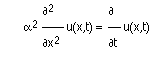To turn this into a recurrence relation we may use the finite difference method, achieving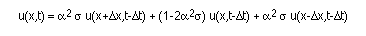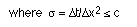The c is a numerical constant, relating the size of the time steps to the size of the object grid. If the quotient is larger than c we loose numerical stability of the method. Abstracting the stencil, we may express the recurrence as a recursive program with the explicit stencil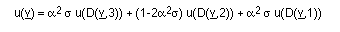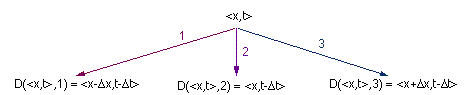The kernel of the program actually compiled follows, where we take into account the boundary conditions (a constant temperature given at the start of the computation)
```h(n) =
if(right_boundary(n) or left_boundary(n)
then h(d(n,2))
else
s*h(d(n,1))
+ (1-2*s)*h(d(n,2))
+ s*h((d(n,3)
```
The actual computation is defined on an integer grid `x int * t int` rather than in real coordinates. The opposite of the stencil becomes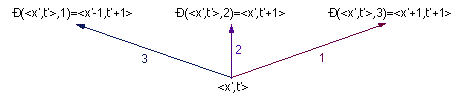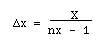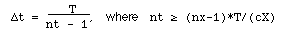where nx is the number of nodes in the discretisation of the object (with length X) in the x-direction, nt is the number of nodes in the discretisation of the simulated time (with length T). The number of time nodes nt is related to the number of space node nx by the given formula.

### Heatflow computation

The following is a sequence of snapshots of the actual computation generated by the compiler. The temperature has been encoded using colours. Initially the rod is hot (yellow), with a constant cold temperature (blue) being applied at the ends. During time the rod cools off from the edges (hues of green).

After sending initial values: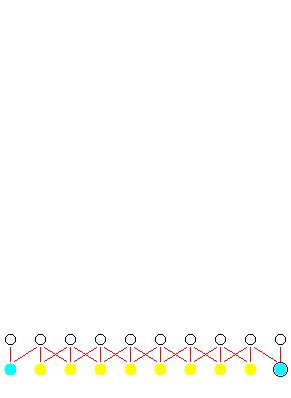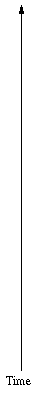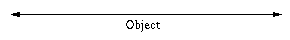While computing: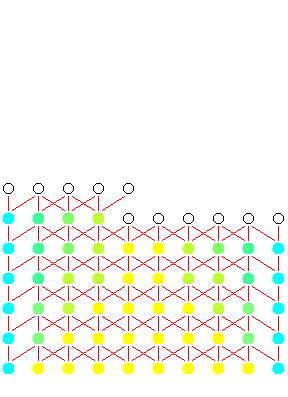Complete computation: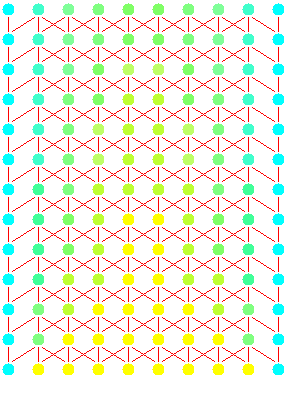You can watch an animated demo of this if your browser supports Java.

In the parallel case all nodes at the same timestep would be computed simultaneously. An animated demo of this has also been prepared as a Java applet.

### Heatflow result

Below the temperature of the object has been plotted against time in a wireframe image. You may view this as a rotatable image written in Java.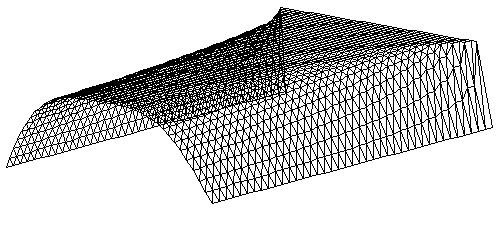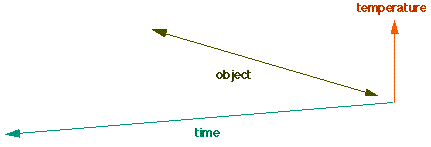This was an example of efficient prototyping of recurrences.
© 1997 Magne Haveraaen, University of Bergen, Norway.